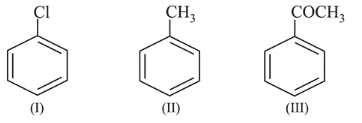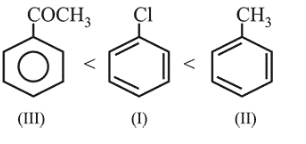# The increasing order of the reactivity of the following

Question:

The increasing order of the reactivity of the following compounds towards electrophilic aromatic substitution reactions is :1. $\mathrm{II}<\mathrm{I}<\mathrm{III}$

2. $\mathrm{III}<\mathrm{II}<\mathrm{I}$

3. $\mathrm{III}<\mathrm{I}<\mathrm{II}$

4. $\mathrm{I}<\mathrm{III}<\mathrm{II}$

Correct Option:

Solution:

$\mathrm{CH}_{3}$ group when bonded to benzene increases the electron density of benzene ring due to $+I$ and hyper conjugation effects. $-\mathrm{Cl}$ group decreases the electron density of benzene ring due to $-\mathrm{I}$ effect, and $-\mathrm{COCH}_{3}$ group strongly decreases the electron density of benzene ring due to $-I$ and $-R$ effects. Therefore, correct increasing order of the given compounds. towards electrophilic aromatic substitution is